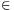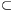Mathematical and Physical Journal
for High Schools
Issued by the MATFUND Foundation
 Already signed up? New to KöMaL?

#Problem A. 569. (October 2012)

A. 569. Call a setinteresting if 2y-xA for all pairs x,yA with x<y. Let a1<a2<...<ak be positive integers (k2) whose greatest common divisor is 1. Prove that if A is an interesting set andthen a1+ak-3A.

Proposed by: Carlos Gustavo T. A. Moreira (Gugu), Rio de Janeiro

(5 pont)

Deadline expired on November 12, 2012.

Solution. We prove a more general statement.

Lemma. If a0<a1<...<ak (k2) are elements of an interesting set A, the greatest common divisor of (a1-a0), (a2-a0), ..., (ak-a0) is 1, and s=ak+a1-a0-3, then {s,s+1,s+2,...}A.

In the particular case a0=0 this implies sA.

We prove the Lemma by induction on (ak-a0).

In the case ak-a02 we have k=2, a1=a0+1 and a2=a0+2, therefore s=a2+a1-a0-3=a0. So, s=a0A, s+1=a1A, és s+2=a2A. The set A is interesting, for every integer x, xA and x+1A impiles x+2=2(x+1)-xA. Hence, s,s+1,s+2,... are all elements of A.

Now suppose that ak-a0>2, and consider the numbers a1,a2,...,ak and 2a1-a0. We will apply the induction hypothesis to these numbers.

Since A is interesting 2a1-a0A. Among 2a1-a0,a1,a2,...,ak the number a1 is the smallest. The greatest common divisor of (a2-a1),...,(ak-a1) and (2a1-a0)-a1) also is 1, due to (ai-a1,(2a1-a0)-a1)=(ai-a1,a1-a0)=(ai-a0,a1-a0).

Case 1: There are at most 2 different values among 2a1-a0,a1,a2,...,ak.

Since a2,...,ak and 2a1-a0 are all greater thatn a1, this means k=2 and 2a1-a0=a2. Then a2-a0=2(a1-a0). The numbers a2-a0 and a1-a0 must be co-primes, so a1-a0=1 and a2-a0=2; this contradicts the assumption ak-a0>2.

Case 2: There are at least 3 distict values among 2a1-a0,a1,a2,...,ak, and 2a1-a0<a2.

Apply the induction hypothesis to b0=a1<b1=2a1-a0<b2=a2<...<bk=ak. (This is allowed by bk-b0=ak-a1<ak-a0.) Let t=bk+b1-b0-3. By the hypothesis. Due to t=ak+(2a1-a0)-a1-3=s, this is what we need.

Case 3: There are at least 3 distict values among 2a1-a0,a1,a2,...,ak, and 2a1-a0>ak.

Apply the induction hypothesis to b0=a1,b1=a2,...,bk-1=ak,bk=2a1-a0. (Not that bk-b0=a1-a0<ak-a0.) Let t=bk+b1-b0-3. By the hypothesis, {t,t+1...}A. By t=(2a1-a0)+a2-a1-3=a2+a1-a0-3s, we have {s,s+1,...}{t,t+1,...}A.

Case 4: There are at least 3 distict values among 2a1-a0,a1,a2,...,ak, and a22a1-a0ak.

Sort the numbers a1,...,ak and 2a1-a0 in increasing order; let the ordered sequence be, where=k or=k-1, b0=a1, b1=a2 és.

Let. Due to b-b0=ak-a1<ak-a0, we can apply the induction hypothesis to get {t,t+1,...}A. Since t=ak+a2-a1-3ak+(2a1-a0)-a1-3=s, we have {s,s+1,...}{t,t+1,...}A.

### Statistics:

 8 students sent a solution. 5 points: Janzer Olivér, Nagy Róbert, Omer Cerrahoglu, Szabó 789 Barnabás. 1 point: 1 student. 0 point: 3 students.

Problems in Mathematics of KöMaL, October 2012